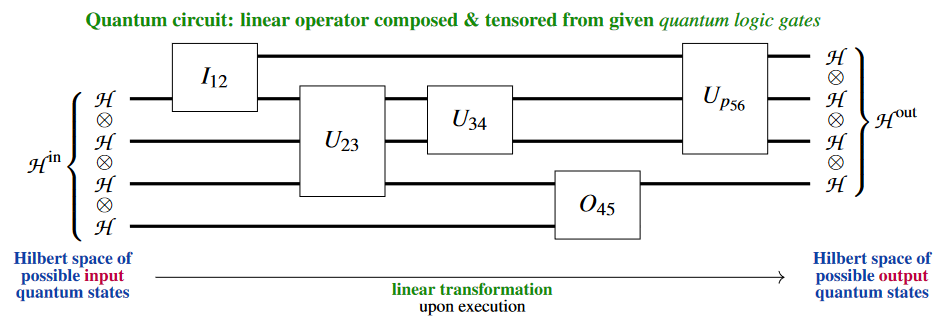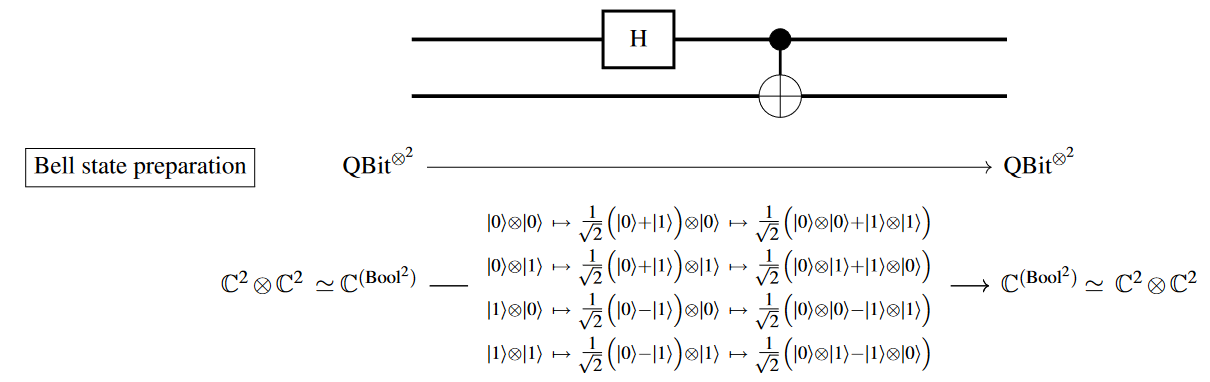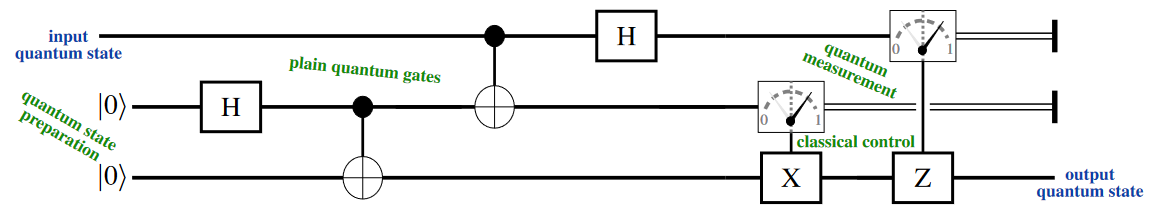# nLab quantum circuit diagram

Contents

### Context

#### Computation

intuitionistic mathematics

### Computability

#### Monoidal categories

monoidal categories

With braiding

With duals for objects

With duals for morphisms

With traces

Closed structure

Special sorts of products

Semisimplicity

Morphisms

Internal monoids

Examples

Theorems

In higher category theory

# Contents

## Idea

Broadly a quantum logic circuit is a logic circuit but in the context of quantum information theory/quantum computation. Concretely, due to the linear logic/linear type theory underlying quantum physics:

In the context of quantum computation, a quantum circuit diagram (originally: “quantum computational network”, Deutsch 1989) is a kind of string diagram (Abramsky & Coecke 2004) in finite-dimensional Hilbert spaces, typically used to express a sequence of low-level quantum gates mapping between spaces of pure quantum states.

A generic pure quantum circuit might look as follows:[figure from Myers et al. 2023]

Here, for instance, the “quantum gate$U_{34}$ is a linear operator on the tensor product Hilbert space $\mathscr{H} \oplus \mathscr{H}$.

Notice that the quantum circuit is understood to be the actual string diagram, up to the usual admissible topological deformations, not just the composite linear transformation which it encodes: The compositeness of the diagram encodes how (e.g. in which order) available quantum gate-operations would be operated on an actual quantum computer, and the composite linear map only encodes the inpute-to-output-specification of the resulting quantum computation. In this sense, quantum circuits constitute a quantum programming language and one also speaks of the “quantum circuit model of quantum computation” (e.g. Nielsen & Chuang 2000, §II.4.6; Miszczak 2011, §3, 2012, §4.3; Beneti & Casati 2018, §3.2).

Accordingly, many existing quantum programming languages are actually domain specific programming languages for quantum circuits, and as such they are often embedded into ambient type theories (for instance Quipper and QWIRE).

Often the basic Hilbert space building block here is taken to be complex 2-dimensional, $\mathscr{H} \,\coloneqq\, \mathbb{C}^2$, in which case one speaks of a quantum circuit on q-bits.

Sometimes all quantum gates involved, and hence also the resulting composite linear maps, are assumed to be invertible, as befits reversible computation by unitary quantum state evolution in quantum mechanics.

But more generally and certainly in the broader context of quantum information theory (such as in formulating the quantum teleportation protocol and similar), quantum circuits are admitted to also contain crucial classical/quantum mechanics-interaction, in the form of:

1. quantum measurement-gates,

2. quantum state preparation-gates,

3. classically controlled quantum gates.

Here quantum measurement, in particular, is not only non-reversible (due to the quantum state collapse involved) but also non-deterministic, meaning that it is not immediately represented by a fixed linear map at all – at least not between the original Hilbert spaces.

One may model the $B$-tuple of projection operators corresponding to the possible set $B$ of measurement outcomes as a linear map between the original Hilbert space $\mathscr{H}$ and its $B$-indexed direct sum (one rare place where this is made explicit, albeit without comment, is Selinger 2004, p. 39):

$\array{ meas & \colon & \mathcal{H} & \xrightarrow{\phantom{--}} & \underset{ b \colon B }{\oplus} \mathcal{H} \\ && \vert \psi \rangle &\mapsto& \underset{b \colon B}{\oplus} \, P_{b} \vert \psi \rangle } \,.$

Therefore, as soon as these three operations crossing the classical/quantum physics-boundary are involved (and made explicit), quantum circuits are no longer just string diagrams in finite-dimensional HilbertSpaces, but something richer, involving besides tensor products also some kind of reflection of direct sums of spaces of quantum states.

For a critical assessment of the (lack of) literature on this issue see Gurevich & Blass 2021.

One approach for handling these more general quantum circuits is (Aharonov, Kitaev & Nisan 1998, Selinger 2004) to understand them, in the tradition on quantum probability theory, as diagrams of “quantum operations” on spaces of mixed quantum states, namely as a kind of string diagrams of completely positive maps between convex spaces of density matrices.

Other approaches for giving categorical semantics for quantum circuits in this general sense have been proposed (and implemented) in the discussion of the quantum programming language Quipper (see the references there). A natural formulation in terms of Quantum Modal Logic in terms of dependent linear type theory is discussed at:

These turn out to be related: From a suitably rich formulation of quantum circuits of pure states with measurement and classical control, the density matrix-formulation may be recovered (…).

At the time of writing (2021) most of the actual programming of experimental quantum computers is conceived through quantum circuit diagrams, while more high-level quantum programming languages are are awaiting the rise of more powerful quantum hardware.

## Examples

### Bell state preparation

In qbit-based quantum computation, the elementary Bell state is usually prepared via the following small quantum circuit consisting of a Hadamard gate followed by a quantum CNOT gate:### Quantum teleportation

The standard (cf. GLRSV13, p. 5) qbit-form of the quantum teleportation-protocol is the following quantum ciruit on qbits (where “$H$” denotes the Hadamard gate, the circles denote the quantum CNOT gates and the boxed pointers denote quantum measurement in the qbit-basis):## Properties

### Quantum circuit diagrams

The notion of quantum gates and quantum circuits acting on pure quantum states originates with:

On quantum circuits for mixed quantum states (with density matrices) and rigorous propsals for the classical/quantum interaction:

Standard textbook accounts:

Standard lecture notes:

• John Preskill, Classical and quantum circuits (pdf), Chapter 5 in: Quantum Computation, lecture notes (web)

• Ryan O’Donnell, Introduction to the Quantum Circuit Model (2015) (pdf)

• Scott Aaronson, §4.2 in: Introduction to Quantum Information Science (2018) [pdf, webpage]

Survey of simulating and designing quantum circuits:

• Alwin Zulehner and Robert Wille: Simulation and Design of Quantum Circuits, in: Reversible Computation: Extending Horizons of Computing. RC 2020 Lecture Notes in Computer Science 12070, Springer (2020) [doi:10.1007/978-3-030-47361-7_3]

The (dagger-compact monoidal) category theoretic formulation is due to:

with exposition and further development in:

For more on this see at finite quantum mechanics in terms of dagger-compact categories.

On quantum circuits as (geodesic, if optimal) computational paths:

Survey, examples, and implementations:

With an eye towards quantum complexity theory:

• Richard Cleve, Section 1.2 in: An Introduction to Quantum Complexity Theory (pdf)

Formal quantum programming language-perspective on quantum circuits:

### Parameterized quantum circuits

Discussion of classically-parameterized quantum circuits:

(…)

Discussion of quantumly-parameterized quantum circuits:

• Evan Peters, Prasanth Shyamsundar, Qarameterized circuits: Quantum parameters for QML [web]

following

• Guillaume Verdon, Jason Pye, Michael Broughton, A Universal Training Algorithm for Quantum Deep Learning [arXiv:1806.09729]

• Prasanth Shyamsundar, Non-Boolean Quantum Amplitude Amplification and Quantum Mean Estimation [arXiv:2102.04975]

### Quantum information theory via String diagrams

#### General

The observation that a natural language for quantum information theory and quantum computation, specifically for quantum circuit diagrams, is that of string diagrams in †-compact categories (see quantum information theory via dagger-compact categories):

On the relation to quantum logic/linear logic:

Early exposition with introduction to monoidal category theory:

Review in contrast to quantum logic:

and with emphasis on quantum computation:

• Jamie Vicary, The Topology of Quantum Algorithms, (LICS 2013) Proceedings of 28th Annual ACM/IEEE Symposium on Logic in Computer Science (2013) 93-102 $[$arXiv:1209.3917, doi:10.1109/LICS.2013.14$]$

Generalization to quantum operations on mixed states (completely positive maps of density matrices):

Textbook accounts (with background on relevant monoidal category theory):

#### Measurement & Classical structures

Formalization of quantum measurement via Frobenius algebra-structures (“classical structures”):

and the evolution of the “classical structures”-monad into the “spider”-diagrams (terminology for special Frobenius normal form, originating in Coecke & Paquette 2008, p. 6, Coecke & Duncan 2008, Thm. 1) of the ZX-calculus:

• Bob Coecke, Ross Duncan, §3 in: Interacting Quantum Observables, in Automata, Languages and Programming. ICALP 2008, Lecture Notes in Computer Science 5126, Springer (2008) $[$doi:10.1007/978-3-540-70583-3_25$]$

• Aleks Kissinger, §§2 in: Graph Rewrite Systems for Classical Structures in $\dagger$-Symmetric Monoidal Categories, MSc thesis, Oxford (2008) $[$pdf, pdf$]$

• Aleks Kissinger, §4 in: Exploring a Quantum Theory with Graph Rewriting and Computer Algebra, in: Intelligent Computer Mathematics. CICM 2009, Lecture Notes in Computer Science 5625 (2009) 90-105 $[$doi:10.1007/978-3-642-02614-0_12$]$

• Bob Coecke, Ross Duncan, Def. 6.4 in: Interacting Quantum Observables: Categorical Algebra and Diagrammatics, New J. Phys. 13 (2011) 043016 $[$arXiv:0906.4725, doi:10.1088/1367-2630/13/4/043016$]$

#### ZX-Calculus

Evolution of the “classical structures”-Frobenius algebra (above) into the “spider”-ingredient of the ZX-calculus for specific control of quantum circuit-diagrams:

• Bob Coecke, Ross Duncan, §3 in: Interacting Quantum Observables, in Automata, Languages and Programming. ICALP 2008, Lecture Notes in Computer Science 5126, Springer (2008) $[$doi:10.1007/978-3-540-70583-3_25$]$

• Aleks Kissinger, Graph Rewrite Systems for Classical Structures in $\dagger$-Symmetric Monoidal Categories, MSc thesis, Oxford (2008) $[$pdf, pdf$]$

• Aleks Kissinger, Exploring a Quantum Theory with Graph Rewriting and Computer Algebra, in: Intelligent Computer Mathematics. CICM 2009, Lecture Notes in Computer Science 5625 (2009) 90-105 $[$doi:10.1007/978-3-642-02614-0_12$]$

• Bob Coecke, Ross Duncan, Interacting Quantum Observables: Categorical Algebra and Diagrammatics, New J. Phys. 13 (2011) 043016 $[$arXiv:0906.4725, doi:10.1088/1367-2630/13/4/043016$]$

Relating the ZX-calculus to braided fusion categories for anyon braiding:

### Quantum programming languages

The first proposal towards formalizing quantum programming languages was:

and early formalization via quantum lambda-calculus invoking linear logic/linear types (cf. Pratt 1992 etc.):

&lbrack;Selinger (2016):&rbrack; When the QPL workshop series was first founded, it was called “Quantum Programming Languages”. One year I wasn’t participating, and while I wasn’t looking they changed the name to “Quantum Physics and Logic” — same acronym!

Back in those days in the early 21st century we were actually trying to do programming languages for quantum computing $[$Selinger & Valiron 2004$]$, but the sad thing is: In those days nobody really cared. $[...]$

Now it’s 15 years later and several of these parameters have changed: There has been a renewed interest, from government agencies and also from companies who are actually building quantum computers. $[...]$.

So now people are working on quantum programming languages again.

Exposition of the general idea of quantum programming languages for classically controlled quantum computation with an eye towards the Quipper-language:

General:

Surveys of existing languages:

• Simon Gay, Quantum programming languages: Survey and bibliography, Mathematical Structures in Computer Science16(2006) (doi:10.1017/S0960129506005378, pdf)

• Sunita Garhwal, Maryam Ghorani , Amir Ahmad, Quantum Programming Language: A Systematic Review of Research Topic and Top Cited Languages, Arch Computat Methods Eng 28, 289–310 (2021) (doi:10.1007/s11831-019-09372-6)

• B. Heim et al., Quantum programming languages, Nat Rev Phys 2 (2020) 709–722 $[$doi:10.1038/s42254-020-00245-7$]$

Quantum programming via quantum logic understood as linear type theory interpreted in symmetric monoidal categories:

The corresponding string diagrams are known in quantum computation as quantum circuit diagrams:

Typed$\;$functional programming languages for quantum computation:

QPL:

• Peter Selinger, Towards a quantum programming language, Mathematical Structures in Computer Science 14 4 (2004) 527–586 $[$doi:10.1017/S0960129504004256, pdf, web$]$

QML:

Quipper:

QWIRE:

CoqQ:

On Q Sharp:

• Kartik Singhal, Kesha Hietala, Sarah Marshall, Robert Rand, Q# as a Quantum Algorithmic Language, Proceedings of Quantum Physics and Logic (2022) $[$arXiv:2206.03532$]$

Quantum programming via dependent linear type theory/indexed monoidal (∞,1)-categories:

specifically with Quipper:

• Manuel A. Serrano, Ricardo Pérez-Castillo, Mario Piattini, Quantum Software Engineering, Springer (2022) $[$doi:10.1007/978-3-031-05324-5$]$
• Linda Anticoli, Carla Piazza, Leonardo Taglialegne, Paolo Zuliani, Towards Quantum Programs Verification: From Quipper Circuits to QPMC, In: Devitt S., Lanese I. (eds.) Reversible Computation. RC 2016, Lecture Notes in Computer Science 9720 Springer (2016) $[$arXiv:1708.06312, doi:10.1007/978-3-319-40578-0_16$]$